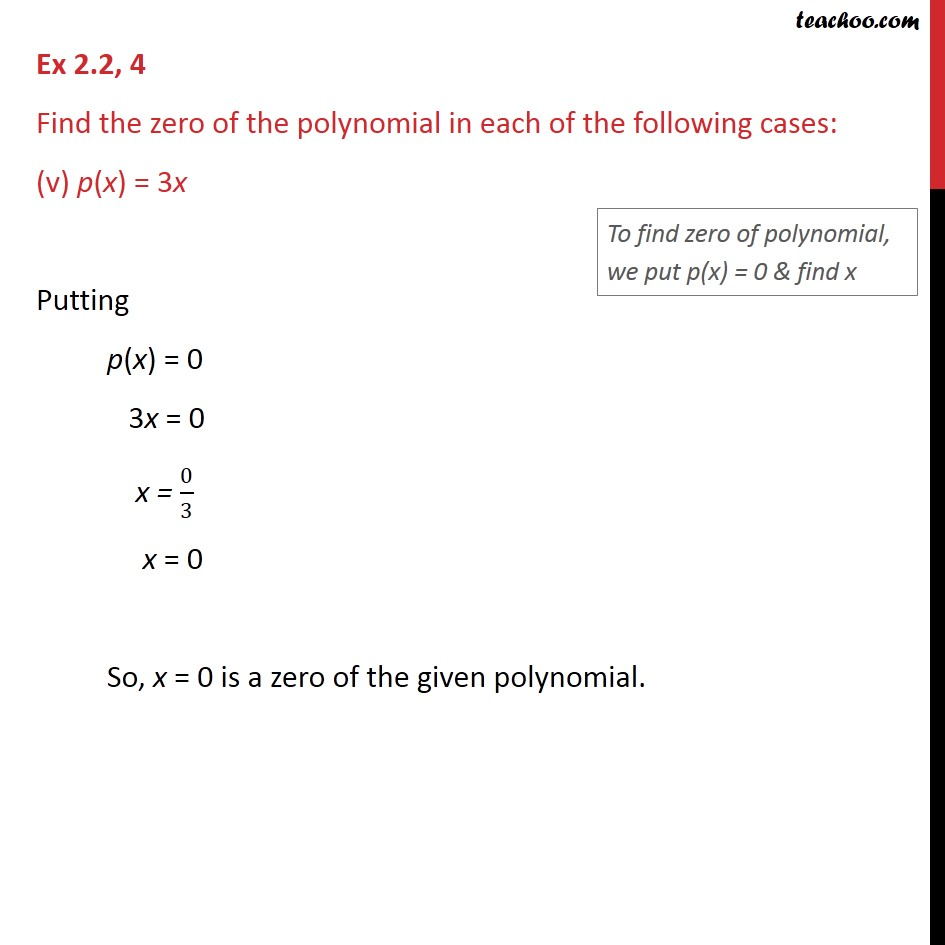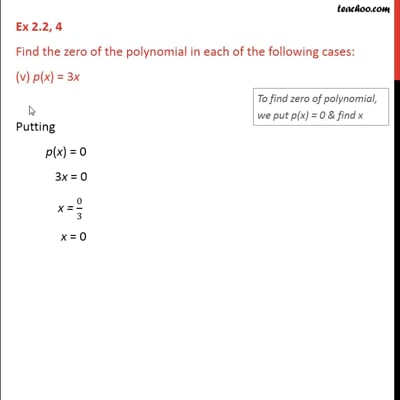Ex 2.2

Chapter 2 Class 9 Polynomials (Term 2)
Serial order wiseThis video is only available for Teachoo black users

### Transcript

Ex 2.2, 4 Find the zero of the polynomial in each of the following cases: (v) p(x) = 3x Putting p(x) = 0 3x = 0 x = 0/3 x = 0 So, x = 0 is a zero of the given polynomial.